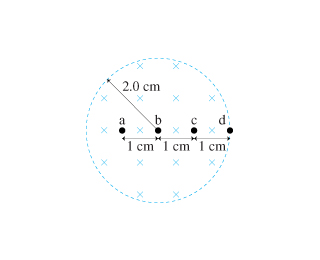# Problem: The magnetic field in the figure is decreasing at the rate of 0.7 T/s.1. What is the magnitude of the acceleration of a proton at rest at point a?2. What is the direction of the acceleration of a proton at rest at point a?a. horizontally to the rightb. downwardc. upwardd. horizontally to the lefte. a = 03. What is the magnitude of the acceleration of a proton at rest at point b? Express your answer as an integer and include the appropriate units.4. What is the direction of the acceleration of a proton at rest at point b?a. horizontally to the rightb. downwardc. upwardd. horizontally to the lefte. a = 05. What is the magnitude of the acceleration of a proton at rest at point c?6. What is the direction of the acceleration of a proton at rest at point c?a. horizontally to the rightb. downwardc. upwardd. horizontally to the lefte. a = 07. What is the magnitude of the acceleration of a proton at rest at point d?8. What is the direction of the acceleration of a proton at rest at point d?a. horizontally to the rightb. downwardc. upwardd. horizontally to the lefte. a = 0

###### FREE Expert Solution

1.

Let's consider an Amperian circle, which passes through and has its center at b.

The motion of the proton induces an emf when placed at the point and is given by:

$\overline{){\mathbf{\epsilon }}{\mathbf{=}}{\mathbf{-}}\frac{\mathbf{d}\mathbf{\varphi }}{\mathbf{d}\mathbf{t}}}$, where Φ is the magnetic flux given by Φ = B•A.

79% (295 ratings)###### Problem Details

The magnetic field in the figure is decreasing at the rate of 0.7 T/s.1. What is the magnitude of the acceleration of a proton at rest at point a?

2. What is the direction of the acceleration of a proton at rest at point a?

a. horizontally to the right

b. downward

c. upward

d. horizontally to the left

e. a = 0

3. What is the magnitude of the acceleration of a proton at rest at point b? Express your answer as an integer and include the appropriate units.

4. What is the direction of the acceleration of a proton at rest at point b?

a. horizontally to the right

b. downward

c. upward

d. horizontally to the left

e. a = 0

5. What is the magnitude of the acceleration of a proton at rest at point c?

6. What is the direction of the acceleration of a proton at rest at point c?

a. horizontally to the right

b. downward

c. upward

d. horizontally to the left

e. a = 0

7. What is the magnitude of the acceleration of a proton at rest at point d?

8. What is the direction of the acceleration of a proton at rest at point d?

a. horizontally to the right

b. downward

c. upward

d. horizontally to the left

e. a = 0

Frequently Asked Questions

What scientific concept do you need to know in order to solve this problem?

Our tutors have indicated that to solve this problem you will need to apply the Ampere's Law concept. If you need more Ampere's Law practice, you can also practice Ampere's Law practice problems.

What professor is this problem relevant for?

Based on our data, we think this problem is relevant for Professor DeJonghe's class at UIC.